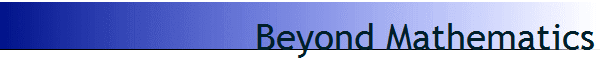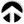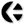### From Mathematics to MetaThematics

The following text is an exploration beyond the boundaries and definition of conventional mathematics.

Conventional mathematics has been defined as being determined by the mathematician.
Its logic is one of control, thus domination, by the mathematician.
Surprise (newness) is eliminated from the equations.
In MetaThematics the interest is the inverse; as mathematics is a creation of our mind; can we calculate how our mind functions?

Mental function has a very crucial discriminating factor: freedom of choice.
Precisely that, what mathematicians have always aimed to eliminate, is what is needed for MetaThematics.
This requires that we explore whatever leeway mathematicians have allowed for, and extend that beyond what they allowed for.

• A brief history of mathematics is called for.

Mathematics started with the calculation by the tax collectors in Egypt: the quickest way to levy taxes was as percentage of whatever was grown on the fields.

• Geometry - earth measuring - focused on determining the surface of differing plots of lands, regardless of their shape, or what was planted.
• Algebra (“Al Jebr”; fractions) focused on the ratio or proportion of the amount of produce, carted off by the taxman.

In combination this led to a focus on operations on amounts; Number and Ratio, are quantity without quality.

• In Geometry the quality is not regarded, and the measurement of the fields needed to be accurate and based on defined units of measurement.
• In Algebra the quality was implied (whatever goods were quantified) and the ratio was a proportion of whatever amount of harvest was being taxed.

In combination algebra and geometry pertained to the proportion of a specific quantity produced by the field, of any given produce.
In practice algebra and geometry have both become abstract operations, done in writing or in the mind, instead of on the land and market.

In the course of time the computations have complexified: ranging from fields to holds of storehouses and ship (volumetric calculations); and multiples thereof.
They have also take into account the change of the harvest over time, and in different regions.
What children now learn in school often has lost touch with the reality of daily life; even if it starts with the adding and subtracting of apples.
What students learn in universities has become even more abstract - with all kinds of clever operations - of which the reason is rarely explained.

Some praise mathematics for its capacity of abstraction; other are praiseful for its precision.
In practice mathematics has become a powerful tool of the mind, and now has many applications.
However it is clear that all those applications are used as example where these forms of mathematics are useful.
And most students of mathematics, in learning formulae, are less and less aware that mathematics is a mental fabrication.

Students are told about various types of Numbers, such as
Real, Imaginary, Logarithmic and Transcendental numbers.
What they often are not being told is that are not dealing with Numbers (quantities) but with specific implied operations (qualities).
Numbers such as i, e, pi, phi are not numbers at all, but specific means of reckoning with relationships.
i and pi, for example, relate to operations in cycles; e and phi are related to reckoning in terms of logarithmic spirals.

• Cycles, or circles, have to do with precise repetition, in which calculations most often pertain to amounts-and-parts of such repetitions.
• Logarithms and spirals have to do with growth; and ratios of increase (acceleration).with an inherent memory function on relative progress.

It will be clear that the differences in number reflect not a change in quantity, but a change in quality: of difference reference systems.
It turns out that the operations with such numbers operate (ex)changes between/within the different reference systems, and their meaning.

It also turns out that the different combinations of operation may bring out ambiguities in the meaning of those implied) reference systems.
It can for example mean that the combination of circles and logarithms leads to the realisation that cycles of different sizes may be related.
It may also become clear that a specific limit, invisible in looking at a circle (which may be scaled at any size), becomes evident by another perspective.
In this manner (via looking at spirals) circles may be seen to be the same as a point; or (at infinity, the open end op a spiral) totally undefined.

Such insights have led to immense amounts of confusions in mathematics.
Mathematicians have habitually attempted to eliminate such instances of confusions.
It has take a very long time before such occurrences were recognised and given a name of their own: singularities.
It took even longer before they became recognised as a fundamental aspect of mathematics (and physics) and special forms of mathematics (Topology) were created to deal with them.

A specific related form of mathematics is known as Dimensional Analysis.
It was formulated by Langhaar, in a book published in 1956.
It focused on the different responses of scale models of e.g. ships, compared to the real size version.
In Dimensional Analysis there is a curious finding that a metric of space, time and mass (energy) has a 'quality' in itself.

Dimensional Analysis defines a Dimensionless Number (which is calculated in a specified manner).
Such a Dimensionless number defines a limit of perception of whatever system is being observed.
It is characteristic that the Dimensionless Number defines a boundary of perception, of observation.
It is important to understand that this critical boundary on the observation depends on the choice of parameters of the observer. !

The observer is thus not simply an observer.
Whatever parameters the observer includes/excludes in the observation determines what, and how, the observer perceives.
This does not only make the observer participant in the observation, but determinant of the outcome.
Important is the realisation that this applies to all observations in physics.

Well-known are the examples of the Dimensionless Numbers of Reynolds, Strouhal, Weber and Mach.
Less well known is that the Dimensionless Number of Mach - the barrier of sound - has an equivalent in the barrier of Light.
All equations of Einstein thus simply define a shift (in limit) of perspective of what can be defined at/as any other limit of observation.
More succinctly we can say that the barriers of sound, light and such are not barriers of physics, but BARRIERS OF CONSCIOUSNESS.

• It is at this level that we can switch from Mathematics to MetaThematics.
• MetaThematics is based on the realisation that mathematicians create and design mathematics.
• Every form of mathematics is created to fulfil a specific purpose.
• Each form of mathematics is yet another shift in perspective, in the sense of Dimensional Analysis.

Different forms of geometry, algebra, topology and logic are simply mental operations.
The emergence of any form of mathematics simply depends on the amount of people that join in, in that perspective.
None of the forms of mathematics are true, nor real: they are but modes of description.
Once you realise this, you can study the taxonomy of the development of different forms of mathematics, to cater top specific needs of specific cultures.

Important is the realisation that mathematics is never about ratio or number; it is always about 'profit and taxes'.
Every form of mathematics is used solely because there is a group of people who benefits from it.
By the lesson of Dimensional Analysis we can see that each form of mathematics reflects a different form of involvement.
By the same lesson we can see that every form of mathematics limits, blinds our perspective on realisation.

For that reason we need to look beyond the possible forms and taxonomies of mathematics.
The relationships between the different forms of mathematics reflect larger developments within a culture.
In that sense we can, e.g. see the transition from number to vector to matrix to N-D (multi-dimensional) Array; as complexity was called for.
Likewise we can see the change from number to cycle to spirals to transcendental numbers when more forms of dynamics were studied.

Whatever form of mathematics you regard, you will see that it allows for, thus reflects, a change in perspective.
In that same way geometry was able to shift from point perspective via line segments to fractals to holograms.
In the opposite a change was seen from the number 1 via 0 (Arab invention) and oo (Cantors realisation) to topologies of different kinds.
The topologies focused on the kind of Singularities Dimensional Analysis also makes explicit: the limits and limitations of mathematics cq. a specific mathematical model.

• This brings us to the need to change from mathematics to MetaThematics.
• It being clear that mathematics is a mental creation, it is clear also it reflects our limitations.
• Mathematics is a form of symbolic operation (symbolic magic; changing our thinking and interactions).
• However Mathematics is not our measure for realisation; mathematics is a result of a process of realisation.

Mathematics deals with abstraction; operations regardless of form of application.
In that same way we need to understand mathematics as an abstract mental operation.
What we see via mathematics is not reality but realisation.
In seeing the singularities - the limits of mathematics - we do not see the limitations of mathematics, but we experience our freedom of choice.

MethaThematics includes the effect of our Involvement.

Every element of mathematics is based on Freedom of Choice.
Mathematics is based on arbitrary choice, and given meaning.
This is the explanation of every axiom: it has no foundation, because it is an arbitrary formulation.
What we seek is not the formulation of reality,
but the basic principles of realisation
.

• MetaThematics is the application of this realisation.
• Mathematics expresses the way we think.
• Singularities represent our Freedom of Choice.
• Together they form the reflection of our way of thinking.

Next: Formal Representation?

#### [Foreword][MetaThematics 00: Introduction][MetaThematics 01: Beyond Mathematics][MetaThematics 02: Toward a Formalism][MetaThematics 03: Symbolic Representation][MetaThematics 04: From Physics to Phasics][MetaThematics 05: The Gravity of Consciousness][MetaThematics 06: The Manifestation of Space][MetaThematics 07: The Art/Act of Involvement][MetaThematics 08: The Formulation of/for Involvement][MetaThematics 09: The g.o.d. experience][MetaThematics 10: The g.o.d. BioLogics][MetaThematics 11: g.o.d. = creation][MetaThematics 12: Primes and Consequences][Welcome] [Core Concepts] [Topics] [Participants] [Publications] [Research] [Projects]